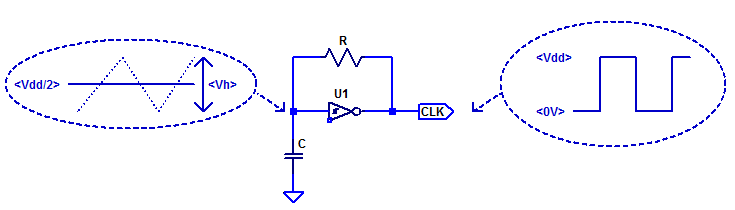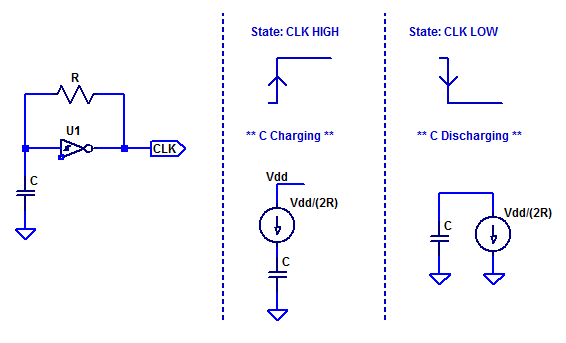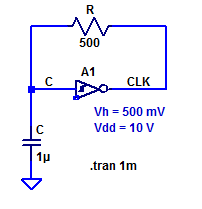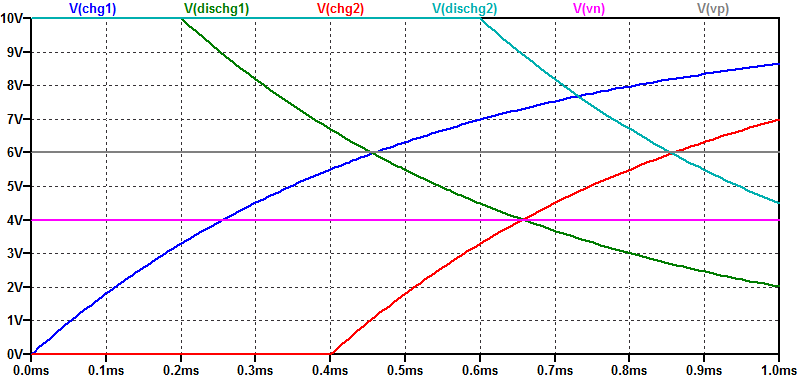# CD40106 Schmitt Trigger Relaxation Oscillator

In a traditional oscillator, oscillation is achieved by providing a loop-gain greater than unity and a phase-shift of $$0^o$$ at some $$f_{osc}$$. However, in the case of a relaxation oscillator, oscillation is achieved by switching between two discrete states. Often a relaxation oscillator is constructed with  a non-linear circuit element which can provide 2  threshold voltage levels to define two discrete states. One non-linear circuit element which is commonly employed in a relaxation oscillator is a schmitt trigger.

Due to the hysteresis developed internal to the schmitt trigger, two trigger thresholds are realized, namely $$V_N$$ and $$V_P$$. Where $$V_N$$ is the negative threshold voltage and $$V_P$$ is the positive threshold voltage. The hysteresis voltage is then,

$$V_H = V_P – V_N$$

Ideally, the thresholds $$V_N$$ and $$V_P$$ would be centered around mid-supply ($$V_{dd}/2$$), however in practice this is only approximately true.

A minimal component count relaxation oscillator is shown in the figure below.Due to the high-gain and hysteresis of a schmitt trigger only two output values are obtained; Vdd for output-high and Ground for output-low. Feedback resistor $$R$$ controls the charge/discharge current of capacitor $$C$$.

## Simplified Theory of Operation

If we consider the hysteresis voltage $$V_H$$ to be small compared to the supply voltage $$V_{dd}$$, then during oscillation, the voltage of capacitor C is approximately $$V_{dd}/2$$. By approximating V(C), we can simplify the charge current to be approximately,

$$I_{chg} =\dfrac{V_{oh} – V_C}{R} = \dfrac{V_{dd} – V_{dd}/2}{R}$$

Equivalently, the discharge current is,

$$I_{dis} =\dfrac{V_C – V_{ol}}{R} = \dfrac{ V_{dd}/2 – 0}{R}$$

Since the average voltage of capacitor C is $$V_{dd}/2$$ the charge/discharge currents are symmetric and equal.

$$I_{chg} = I_{dis} =\dfrac{ V_{dd}}{2R}$$

We can analyze the two discrete states independently to determine the period of oscillation. A simplified schematic and state model of the oscillator is shown in the figure below.When the output transitions high the capacitor begins charging. Since we assumed $$V_H$$ to be small compared to $$V_{dd}$$ the current is approximately constant. Hence, we can simplify R as a constant current source.

When power to the circuit is initially applied, the capacitor is completely discharged. Since U1 is inverting, its output is high and capacitor C begins charging. The figure below summarizes the sequence of events that occur during oscillation.(1)  Start-up, capacitor C begins charging until its value reaches the the threshold voltage $$V_P$$.

(2)  With V(c) greater than $$V_P$$ the output transitions low, hysteresis internal to the schmitt trigger shifts the threshold to $$V_N$$.

(3)  With the output low, capacitor C begins discharging.

(4)  Capacitor C discharges below threshold voltage $$V_N$$ causing the output to transition high. Hysteresis internal to the schmitt trigger shifts the threshold voltage to $$V_P$$

(5)  Capacitor C begins charging.

(…)  Process repeats indefinitely.

## Period of Oscillation – Simple Model

For the simplified model of the relaxation oscillator, we can determine the period of oscillation based on the charge equation of a capacitor,

$$Q = CV$$

For the charging case we know the capacitor must charge from $$V_N$$ to $$V_P$$, a total voltage of $$V_H$$. We can solve the charge required as,

$$\Delta Q = C V_H$$

The time needed to accumulate $$\Delta Q$$ coulombs of charge on capacitor C is then,

$$\Delta t = \dfrac{ \Delta Q}{ I_{chg} }$$

Since the charge/discharge currents are equal the charge/discharge periods are then,

$$t_{chg} = \dfrac{CV_h}{I_{chg}},\;\;\;t_{dis} = \dfrac{CV_h}{I_{dis}}$$

From the assumptions above of small $$V_H$$ at mid-supply the charge/discharge current is,

$$I_{chg} \simeq I_{dis} = \dfrac{V_{dd}/2}{R}$$

Finally, the total period of oscillation is then,

$$T_{osc} = t_{chg} + t_{dis} = 4 RC \dfrac{ V_h}{V_{dd}}$$

Equivalently, the frequency of oscillation is,

$$f_{osc} = 1/T_{osc} = \dfrac{ V_{dd}}{4RCV_h}$$

As an example consider the following relaxation oscillator,The period of oscillation for the sample circuit above is then approximately,

$$T_{osc} = 4 (500 \; \Omega)( 1\; \mu\text{F} )\left( \dfrac{ 0.5\;\text{V}}{10\;\text{V}}\right) = 100 \; \mu\text{s}$$

A spice transient simulation of the sample oscillator is shown in the figure below.

After start-up the period of oscillation is approximately 100 $$\mu s$$. The charge/discharge current is not constant, but rather, it varies from 9.5 mA to 10.5 mA over a charge/discharge cycle.

## Period of Oscillation – Complex Model

Instead of assuming the charge/discharge current is constant and symmetric we can instead analyze the period of oscillation based on the step response of an RC circuit. The figure below depicts several RC charge/discharge cycles transitioning through threshold voltages $$V_N$$ and $$V_P$$.The general solution for the step response of an RC circuit is the following,

$$V_C(t) = V_C(0) + \left( V_C(\infty) – V_C(0) \right)\left( 1 – e^{-t/\tau} \right)$$

### Charge Time

During the capacitor charging cycle, the capacitor begins with a \voltage (V_N\) and terminates charging at voltage $$V_P$$. If the charge cycle was not terminated (i.e. not oscillating) the steady-state value would reach power supply voltage $$V_{dd}$$. Thus we have the following boundary conditions,

$$V_C(0) = V_P, \;\;\; V_C(\infty) = V_{dd}$$

Substituting the boundary conditions for the charge cycle into the general solution yields,

$$V_C(t) = V_N + \left( V_{dd} – V_N \right)\left( 1 – e^{-t/\tau} \right)$$

Where,
$$\tau = RC$$
is the RC time-constant of the circuit.

We can solve for the charge time by solving for when for when the capacitor reaches a potential of $$V_P$$ as,
\begin{align*}
V_C(t_c) &= V_P \\
V_P &= V_N + \left( V_{dd} – V_N \right)\left( 1 – e^{-t_c/\tau} \right)\\
V_P – V_N – V_{dd} + V_N &= \left( V_{dd} – V_N \right)\left( – e^{-t_c/\tau} \right)\\
\dfrac{ V_{dd} – V_P }{V_{dd} – V_N } &= e^{-t_c/\tau} \\
\log \left( \dfrac{ V_{dd} – V_P }{V_{dd} – V_N } \right) &= \dfrac{-t_c}{\tau}
\end{align*}

Finally, the time duration of the charge cycle is,

$$t_c = \tau \log\left( \dfrac{V_{dd} – V_N }{ V_{dd} – V_P } \right)$$

### Discharge Time

During the discharge cycle the capacitor discharges from threshold voltage $$V_P$$ to threshold voltage $$V_N$$. If the capacitor was left to fully discharge the final steady-state value would be 0 VDC. With boundary conditions,

$$V_C(0) = V_P, \;\;\; V_C(\infty) = 0$$

Substituting the boundary conditions into the general solution yields,
\begin{align*}
V_C(t) &= V_P + \left( 0 – V_P \right)\left( 1 – e^{-t/\tau} \right) \\
V_C(t) &= V_P \left( e^{-t/\tau} \right)
\end{align*}

Solving for when the capacitor discharges to potential $$V_N$$,

\begin{align*}
V_C(t_d) &= V_N \\
V_N &= V_P \left( e^{-t_d/\tau} \right) \\
\log \left( \dfrac{V_N}{V_P} \right) &= \dfrac{-t_d}{\tau}
\end{align*}

The time duration for the discharge cycle is then,

$$t_d = \tau \log \left( \dfrac{V_P}{V_N} \right)$$

### Period of Oscillation

Knowing the time duration of both the charge and discharge cycles, the period of oscillation is then,

$$T = t_c + t_d = \tau \log\left( \dfrac{V_{dd} – V_N }{ V_{dd} – V_P } \right) + \tau \log \left( \dfrac{V_P}{V_N} \right)$$

After some algebra, the final solution is,

$$T = RC \log\left( \dfrac{ \left(V_{dd} – V_N\right)V_P }{ \left(V_{dd} – V_P\right)V_N } \right)$$

Which is identical to the solution presented in the CD40106 datasheet, as shown in the figure below.## 1 thought on “CD40106 Schmitt Trigger Relaxation Oscillator”

This site uses Akismet to reduce spam. Learn how your comment data is processed.